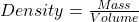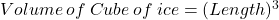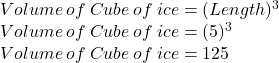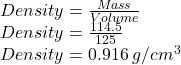## (1) A cube of ice has side length of 5cm. The mass of the cube of ice is 114.5g. Find the density of ice. Give your answer

Question

(1) A cube of ice has side length of 5cm.
The mass of the cube of ice is 114.5g.
Find the density of ice.

in progress 0
3 months 2021-08-12T23:25:19+00:00 2 Answers 0 views 0

Density of Ice = 0.916 g/cm^3

Step-by-step explanation:

Length of Cube of ice = 5 cm

Mass of Cube = 114.5 g

We need to find density of ice.

The formula used to find density of ice is:Before. finding density, we need to find volume of cube of ice.

The formula used for finding volume is:We have Length = 5

So, Volume will be:We get Volume of cube of ice = 125

Now finding densitySo, We get Density = 0.916 g/cm^3